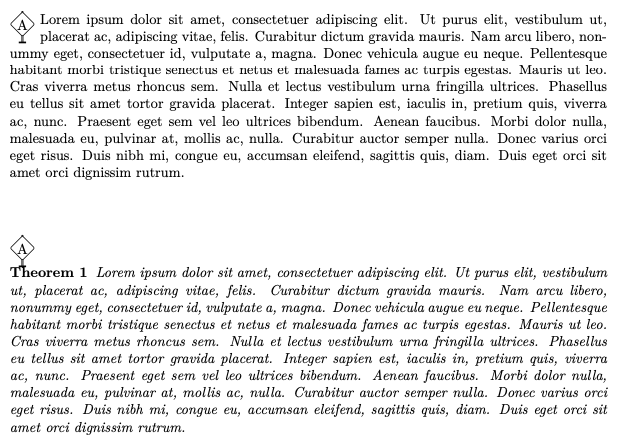# #StackBounty: #tikz-pgf #theorems How to make the theorem environment flow around campa's code for TeXbook's bend symbol?

### Bounty: 50

This is a follow up question to this comment. How can I make campa’s code for TeXbook’s bend symbol work with the `theorem` environment?

Consider the following LaTeX code which I saved in a file called `test.tex`:

``````documentclass{scrartcl}
usepackage{tikz}
newenvironment{mydanger}{%
sbox0{%
begin{tikzpicture}[scale=.3]
draw[rounded corners=.1] (-.05,-1.5)--++(0,2.55)--++(.1,0)--++(0,-2.55);
draw[fill=white,rounded corners=1] (0,1)--(1,0)--(0,-1)--(-1,0)--cycle;
draw[very thick](-.3,-1.5)--(.3,-1.5);
node at (0,0) {small#1};
end{tikzpicture}
}%       this space ^^^ is here for a reason!
parmedskip  noindent
hangindentwd0
parindenthangindent
hangafter=-2
setbox0=hbox to0pt{hsslower3exbox0}%
dp0=0pt
box0
small
ignorespaces
}{parsmallskip}
newtheorem{theorem}{Theorem}
usepackage{lipsum}
begin{document}
begin{mydanger}{A}
lipsum
end{mydanger}

vspace{1cm}

begin{mydanger}{A}
begin{theorem}
lipsum
end{theorem}
end{mydanger}
end{document}
``````

When I run this code with `lualatex test`, the following output is typeset:I’d like the theorem paragraph to flow around the bend symbol like the regular paragraph above it.

Important requirement

The solution must be consistent with the possibility of adding margin notes via `marginpar` inside the theorem. Therefore, for instance, a solution that encapsulates the theorem inside an invisible `tcolorbox` will not be an acceptable solution; see here.

Get this bounty!!!

This site uses Akismet to reduce spam. Learn how your comment data is processed.